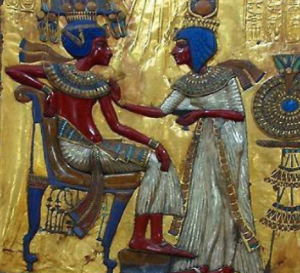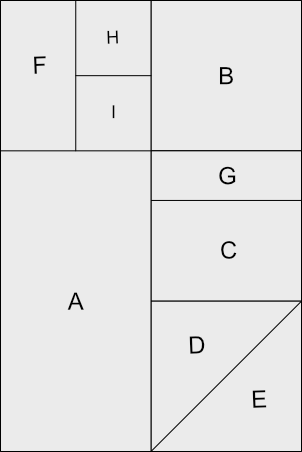# The Pharaoh's problem

## Set up:The Pharaoh draws a rectangle in a clay tablet and decrees nine houses (A - I) shall grow grain, in their assigned areas. Where upoon, the grain shall be collected and stored in the Pharaoh's grain houses for the use of the dynasty.

As chief treasurer, your task is to record the size of the areas planted. So you ask the Pharaoh, the area of the rectangle. The Pharaoh, who always tries to stump you, thinks she can, if she uses a prime number for the area so she answers:

The area of the retangle is 17 square units.

Find the area of all the shapes in the rectangle.

• Assume proportions are as they appear. Which would mean ...
• The area of B = the area of (G + C);
• The area of B = the area of (D + E);
• The area of A = the area of 2(F + H + I)
• The area of 3G = the area of B;
• The area of 4H = the area of B
• The area of D = the area of E

The clay tablet:A =

B =

C =

D =

E =

F =

G =

H =

I =

Brain buster: What are the dimensions for each shape?

Hint 1:

Think of each shape's area as a proportion of the area of the whole rectangle.

Hint 2:

What proportion of the area of the rectangle is the area of B?

Hint 3:

What proportion, of the area of the rectangle, is each of the shapes?

Hint 4:

Make a list with each shape and its fractional part of the whole rectangle?

Listed from largest to smallest.

Shape Fraction of whole rectangle Fraction of one Equivalent fractions as 72'nds
A 2/6 or 1/3 1/3 24/72
B 1/6 1/6 12/72
C 2/3 of 1/6 2/18 8/72
D 1/2 of 1/6 1/12 6/72
E 1/2 of 1/6 1/12 6/72
F 1/2 of 1/6 1/12 6/72
G 1/3 of 1/6 1/18 4/72
H 1/4 of 1/6 1/24 3/72
I 1/4 of 1/6 1/24 3/72
Common denominator for
3, 6, 12, 18, 24
is - 72
Sum of one or 72/72

Hint: 5

Now use the fractional parts of the whole rectangle to find the fractional part of 17 square units.

Shape Fraction of 17 square units Find an equivalent fraction as 72'nds Find an equivalent fraction as 72'nds
A 17/3 17 * 24/24 = 408 / 72 408 / 72 =
B 17/6 17 * 12/12 = 204 / 72 204 / 72 =
C 2/3 * 17/6 = 34/18 34 * 4/4 = 136 / 72 136 / 72 =
D 17/12 17 * 6/6 = 102 / 72 102 / 72 =
E 17/12 17 * 6/6 = 102 / 72 102 / 72 =
F 17/12 17 * 6/6 = 102 / 72 102 / 72 =
G 17/18 17 * 4/4 = 68 / 72 68 / 72 =
H 17/24 17 * 3/3 = 51 / 72 51 / 72 =
I 17/24 17 * 3/3 = 51 / 72 51 / 72 =
Sum is 1,224 / 72 = 17 square units Sum is?

Top